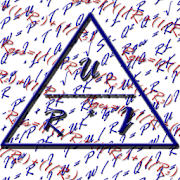# Electrician AppEveryone
60
Electrician App - is a good companion for an electrician or electronics engineer in training, on the job or for hobby electricians and handymen.

Electrician App contains the most important formulas in the field of electrical engineering. Easy to use, available in 3 languages​​: English, German and Russian.

Features of Electrician App:

Flow divider.
Calculation of up to twenty resistors (parallel).
Calculation of up to twenty resistors (in series).
Calculation of resistors (mixed).
Calculation of current, charge and time.
Calculation of work, time and power.
Calculation of line resistance.
Calculation of current, voltage, power and resistance.
Calculating the voltage drop on the line.
Calculating the maximum length of the conductor.
Calculation of the conductor cross-section.
Calculation of real, reactive, apparent power at AC.
Calculation of real, reactive, apparent power for three-phase.
Calculation of primary voltage, secondary voltage, primary windings, secondary windings of a transformer.
Calculating the cross section and diameter.
Calculation of the current density.
Calculation of cos φ.
Calculation of sin φ.

For the accuracy of the calculation, we assume no liability.

Forget complicated formulas and calculation methods, specify a value-known and Electrician App returns the result. Several updates and new formulas more languages ​​are planned in the near future.

Electrician App is optimized and improved by us and as for we depend on your help.

Collapse

Review Policy
4.2
60 total
5
4
3
2
1

## What's New

Total resistance (parallel)
Total resistance (in series)
Total resistance (mixed)
Collapse

Updated
December 10, 2014
Size
2.7M
Installs
10,000+
Current Version
3.5
Requires Android
1.6 and up
Content Rating
Everyone
Permissions
Offered By
fellex
Developer
Alexander Funtikov Höhenring 91A 53913 Swisttal